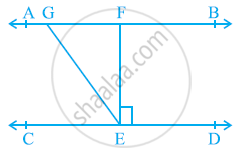Share

# In the given figure, If AB || CD, EF ⊥ CD and ∠GED = 126º, find ∠AGE, ∠GEF and ∠FGE. - CBSE Class 9 - Mathematics

ConceptLines Parallel to the Same Line

#### Question

In the given figure, If AB || CD, EF ⊥ CD and ∠GED = 126º, find ∠AGE, ∠GEF and ∠FGE.#### Solution

It is given that,

AB || CD

EF ⊥ CD

∠GED = 126º

⇒ ∠GEF + ∠FED = 126º

⇒ ∠GEF + 90º = 126º

⇒ ∠GEF = 36º

∠AGE and ∠GED are alternate interior angles.

⇒ ∠AGE = ∠GED = 126º

However, ∠AGE + ∠FGE = 180º (Linear pair)

⇒ 126º + ∠FGE = 180º

⇒ ∠FGE = 180º − 126º = 54º

∴ ∠AGE = 126º, ∠GEF = 36º, ∠FGE = 54º

Is there an error in this question or solution?

#### APPEARS IN

NCERT Solution for Mathematics Class 9 (2018 to Current)
Chapter 6: Lines and Angles
Ex. 6.20 | Q: 3 | Page no. 105

#### Video TutorialsVIEW ALL 

Solution In the given figure, If AB || CD, EF ⊥ CD and ∠GED = 126º, find ∠AGE, ∠GEF and ∠FGE. Concept: Lines Parallel to the Same Line.
S